Data Structures and Algorithms with Object-Oriented Design Patterns in C### Applications

Consider the following expression: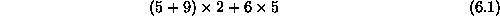In order to determine the value of this expression, we first compute the sum 5+9 and then multiply that by 2. Then we compute the product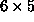and add it to the previous result to get the final answer. Notice that the order in which the operations are to be done is crucial. Clearly if the operations are not done in the correct order, the wrong result is computed.

The expression above is written using the usual mathematical notation. This notation is called infix  notation. What distinguishes this notation is the way that expressions involving binary operators are written. A binary operator  is an operator which has exactly two operands, such as + and. In infix notation, binary operators appear in between their operands.

Another characteristic of infix notation is that the order of operations is determined by operator precedence . For example, the(multiplication) operator has higher precedence than does the + (addition) operator. When an evaluation order is desired that is different from that provided by the precedence, parentheses , ``('' and ``)'', are used to override precedence rules. An expression in parentheses is evaluated first.

As an alternative to infix, the Polish logician Jan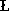ukasiewicz  introduced notations which require neither parentheses nor operator precedence rules. The first of these, the so-called Polish notation , places the binary operators before their operands. For Equationwe would write: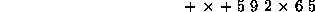This is also called prefix notation, because the operators are written in front of their operands.

While prefix notation is completely unambiguous in the absence of parentheses, it is not very easy to read. A minor syntactic variation on prefix is to write the operands as a comma-separated list enclosed in parentheses as follows: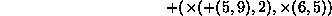While this notation seems somewhat foreign, in fact it is precisely the notation that is used for static method calls in C#:

`Plus(Times(Plus(5,9) ,2), Times(6,5));`

The second form ofukasiewicz notation is the so-called Reverse-Polish notation  (RPN ). Equationis written as follows in RPN: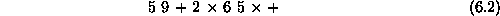This notation is also called postfix notation for the obvious reason--the operators are written after their operands.

Postfix notation, like prefix notation, does not make use of operator precedence nor does it require the use of parentheses. A postfix expression can always be written without parentheses that expresses the desired evaluation order. For example, the expression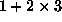, in which the multiplication is done first, is written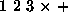; whereas the expression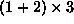is written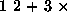.Copyright © 2001 by Bruno R. Preiss, P.Eng. All rights reserved.